# hilbert

Discrete-time analytic signal using Hilbert transform

## Syntax

``x = hilbert(xr)``
``x = hilbert(xr,n)``

## Description

example

````x = hilbert(xr)` returns the analytic signal, `x`, from a real data sequence, `xr`. If `xr` is a matrix, then `hilbert` finds the analytic signal corresponding to each column.```

example

````x = hilbert(xr,n)` uses an `n`-point fast Fourier transform (FFT) to compute the Hilbert transform. The input data is zero-padded or truncated to length `n`, as appropriate.```

## Examples

collapse all

Define a sequence and compute its analytic signal using `hilbert`.

```xr = [1 2 3 4]; x = hilbert(xr)```
```x = 1×4 complex 1.0000 + 1.0000i 2.0000 - 1.0000i 3.0000 - 1.0000i 4.0000 + 1.0000i ```

The imaginary part of `x` is the Hilbert transform of `xr`, and the real part is `xr` itself.

`imx = imag(x)`
```imx = 1×4 1 -1 -1 1 ```
`rex = real(x)`
```rex = 1×4 1 2 3 4 ```

The last half of the discrete Fourier transform (DFT) of `x` is zero. (In this example, the last half of the transform is just the last element.) The DC and Nyquist elements of `fft(x)` are purely real.

`dft = fft(x)`
```dft = 1×4 complex 10.0000 + 0.0000i -4.0000 + 4.0000i -2.0000 + 0.0000i 0.0000 + 0.0000i ```

The `hilbert` function finds the exact analytic signal for a finite block of data. You can also generate the analytic signal by using an finite impulse response (FIR) Hilbert transformer filter to compute an approximation to the imaginary part.

Generate a sequence composed of three sinusoids with frequencies 203, 721, and 1001 Hz. The sequence is sampled at 10 kHz for about 1 second. Use the `hilbert` function to compute the analytic signal. Plot it between 0.01 seconds and 0.03 seconds.

```fs = 1e4; t = 0:1/fs:1; x = 2.5 + cos(2*pi*203*t) + sin(2*pi*721*t) + cos(2*pi*1001*t); y = hilbert(x); plot(t,real(y),t,imag(y)) xlim([0.01 0.03]) legend('real','imaginary') title('hilbert Function')```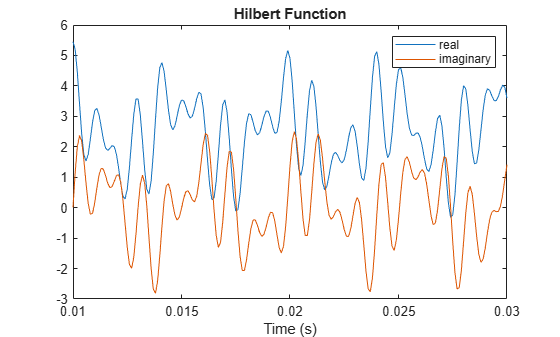Compute Welch estimates of the power spectral densities of the original sequence and the analytic signal. Divide the sequences into Hamming-windowed, nonoverlapping sections of length 256. Verify that the analytic signal has no power at negative frequencies.

```pwelch([x;y].',256,0,[],fs,'centered') legend('Original','hilbert')```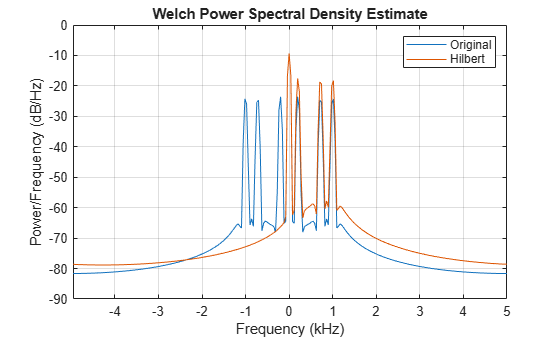Use the `designfilt` function to design a 60th-order Hilbert transformer FIR filter. Specify a transition width of 400 Hz. Visualize the frequency response of the filter.

```fo = 60; d = designfilt('hilbertfir','FilterOrder',fo, ... 'TransitionWidth',400,'SampleRate',fs); freqz(d,1024,fs)```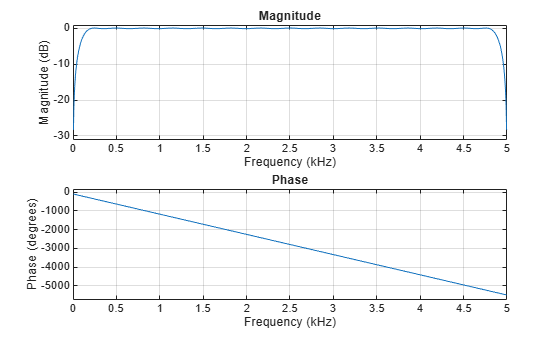Filter the sinusoidal sequence to approximate the imaginary part of the analytic signal.

`hb = filter(d,x);`

The group delay of the filter, `grd`, is equal to one-half the filter order. Compensate for this delay. Remove the first `grd` samples of the imaginary part and the last `grd` samples of the real part and the time vector. Plot the result between 0.01 seconds and 0.03 seconds.

```grd = fo/2; y2 = x(1:end-grd) + 1j*hb(grd+1:end); t2 = t(1:end-grd); plot(t2,real(y2),t2,imag(y2)) xlim([0.01 0.03]) legend('real','imaginary') title('FIR Filter')```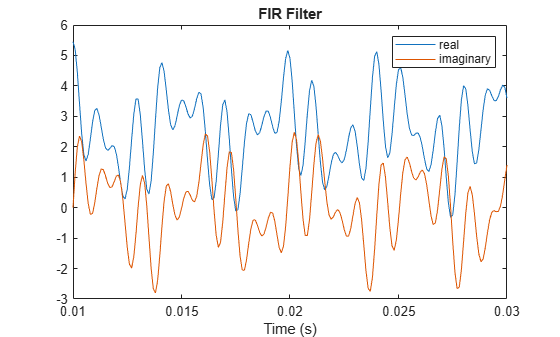Estimate the power spectral density (PSD) of the approximate analytic signal and compare it to the `hilbert` result.

```pwelch([y;[y2 zeros(1,grd)]].',256,0,[],fs,'centered') legend('hilbert','FIR Filter')```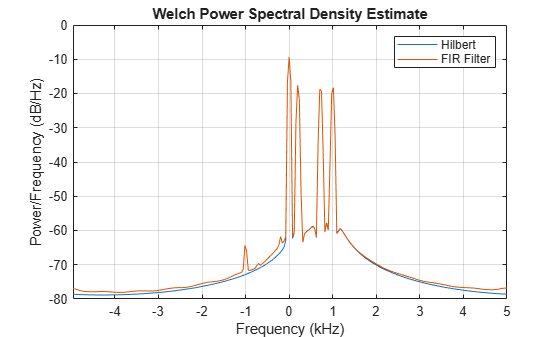## Input Arguments

collapse all

Input signal, specified as a real-valued vector or matrix. If `xr` is complex, then `hilbert` ignores its imaginary part.

Example: `sin(2*pi*(0:15)/16)` specifies one period of a sinusoid.

Example: `sin(2*pi*(0:15)'./[16 8])` specifies a two-channel sinusoidal signal.

Data Types: `single` | `double`

DFT length, specified as a positive integer scalar.

Data Types: `single` | `double`

## Output Arguments

collapse all

Analytic signal, returned as a vector or matrix.

collapse all

### Analytic Signal

`hilbert` returns a complex helical sequence, sometimes called the analytic signal, from a real data sequence.

The analytic signal x = xr + jxi has a real part, xr, which is the original data, and an imaginary part, xi, which contains the Hilbert transform. The imaginary part is a version of the original real sequence with a 90° phase shift. Sines are therefore transformed to cosines, and conversely, cosines are transformed to sines. The Hilbert-transformed series has the same amplitude and frequency content as the original sequence. The transform includes phase information that depends on the phase of the original.

The Hilbert transform is useful in calculating instantaneous attributes of a time series, especially the amplitude and the frequency. The instantaneous amplitude is the amplitude of the complex Hilbert transform; the instantaneous frequency is the time rate of change of the instantaneous phase angle. For a pure sinusoid, the instantaneous amplitude and frequency are constant. The instantaneous phase, however, is a sawtooth, reflecting how the local phase angle varies linearly over a single cycle. For mixtures of sinusoids, the attributes are short term, or local, averages spanning no more than two or three points. See Hilbert Transform and Instantaneous Frequency for examples.

Reference  describes the Kolmogorov method for minimum phase reconstruction, which involves taking the Hilbert transform of the logarithm of the spectral density of a time series. The toolbox function `rceps` performs this reconstruction.

## Algorithms

The analytic signal for a sequence `xr` has a one-sided Fourier transform. That is, the transform vanishes for negative frequencies. To approximate the analytic signal, `hilbert` calculates the FFT of the input sequence, replaces those FFT coefficients that correspond to negative frequencies with zeros, and calculates the inverse FFT of the result.

`hilbert` uses a four-step algorithm:

1. Calculate the FFT of the input sequence, storing the result in a vector `x`.

2. Create a vector `h` whose elements `h(i)` have the values:

• 1 for `i` = 1, `(n/2)+1`

• 2 for `i` = 2, 3, ... , `(n/2)`

• 0 for `i` = `(n/2)+2`, ... , `n`

3. Calculate the element-wise product of `x` and `h`.

4. Calculate the inverse FFT of the sequence obtained in step 3 and returns the first `n` elements of the result.

This algorithm was first introduced in . The technique assumes that the input signal, `x`, is a finite block of data. This assumption allows the function to remove the spectral redundancy in `x` exactly. Methods based on FIR filtering can only approximate the analytic signal, but they have the advantage that they operate continuously on the data. See Single-Sideband Amplitude Modulation for another example of a Hilbert transform computed with an FIR filter.

 Claerbout, Jon F. Fundamentals of Geophysical Data Processing with Applications to Petroleum Prospecting. Oxford, UK: Blackwell, 1985.

 Marple, S. L. “Computing the Discrete-Time Analytic Signal via FFT.” IEEE® Transactions on Signal Processing. Vol. 47, 1999, pp. 2600–2603.

 Oppenheim, Alan V., Ronald W. Schafer, and John R. Buck. Discrete-Time Signal Processing. 2nd Ed. Upper Saddle River, NJ: Prentice Hall, 1999.

﻿